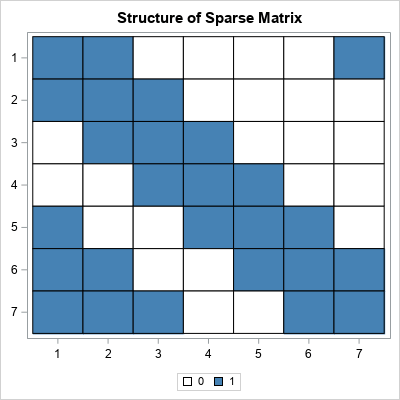# Visualize the structure of a sparse matrix

June 22, 2020
By

 This post was kindly contributed by The DO Loop - go there to comment and to read the full post.

Sometimes in matrix computations, it is important to display the nonzero elements of a matrix. This can be useful for visualizing the structure of a sparse matrix (one that has many zeros) and it is also useful when describing a matrix algorithm (such as Gaussian elimination) that introduces zeros at each step of the algorithm.

Let A be the matrix you want to visualize.
You can visualize the nonzero elements of the matrix A in a graph or in a table:

• Use a custom SAS format that prints an asterisk (*) for nonzero values and a blank for zero values.
• Create a discrete heat map of the binary matrix A^=0, which replaces the nonzero cells of A with the value 1.

### A text display method

For small matrices, you can use a custom SAS format to print the matrix.
The format is defined by using PROC FORMAT. This format displays a star (*) for nonzero values and displays a blank for zero values:

``` proc format; value SparseFmt 0 = " " /* display blank instead of 0 */ other = "*"; /* display '*' instead of number */ run;   proc iml; A = {1.0 0.2 0.0 0.0 0.0 0.0 1e-6, 0.2 1.0 0.2 0.0 0.0 0.0 0.0, 0.0 0.2 1.0 0.2 0.0 0.0 0.0, 0.0 0.0 0.2 1.0 0.2 0.0 0.0, 1e-6 0.0 0.0 0.2 1.0 0.2 0.0, 2e-6 1e-6 0.0 0.0 0.2 1.0 0.2, 3e-6 2e-6 1e-6 0.0 0.0 0.2 1.0 };   /* use a custom format to print * or blank in each cell */ print A[format=SparseFmt.]; ```You can see the banded structure of the matrix and also see that elements near the corners are nonzero.
This textual visualization is concise and is used in textbooks about numerical linear algebra.

### A graphical method

For larger matrices, a graphical method is probably better. You can form the binary logical matrix B=(A^=0) and then plot B by using a two-color heat map. In the SAS/IML language, you can use the HEATMAPDISC call to create a discrete heat map of a matrix. I like to use white for the zeroes. The following SAS/IML statements define a 7 x 7 matrix and use a discrete heat map to display the nonzero values:

``` /* discrete heat map of binary matrix A^=0 */ ods graphics / width=400px height=400px; call heatmapdisc(A^=0) colorramp={white steelblue} title="Discrete heat map of binary matrix"; ```The information in the heat map is identical to the printed display. However, for large matrices, the heat map is easier to view. As shown, you can also use the ODS GRAPHICS statement to control the dimensions of the graph. So, for example, if you are visualizing a square matrix, you can make the graph square.

The post Visualize the structure of a sparse matrix appeared first on The DO Loop.This post was kindly contributed by The DO Loop - go there to comment and to read the full post.

Tags: , ,

## Welcome!

SAS-X.com offers news and tutorials about the various SAS® software packages, contributed by bloggers. You are welcome to subscribe to e-mail updates, or add your SAS-blog to the site.## Recent Posts

SAS and all other SAS Institute Inc. product or service names are registered trademarks or trademarks of SAS Institute Inc. in the USA and other countries. ® indicates USA registration.